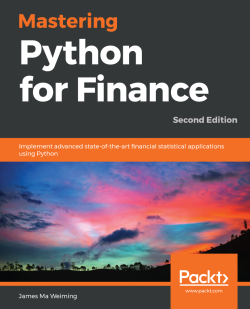### Mastering Python for Finance - Second Edition4.3 (4 reviews total)
By James Ma Weiming
• Constantly updated with 100+ new titles each month
• Breadth and depth in over 1,000+ technologies

The second edition of Mastering Python for Finance will guide you through carrying out complex financial calculations practiced in the industry of finance by using next-generation methodologies. You will master the Python ecosystem by leveraging publicly available tools to successfully perform research studies and modeling, and learn to manage risks with the help of advanced examples.

You will start by setting up your Jupyter notebook to implement the tasks throughout the book. You will learn to make efficient and powerful data-driven financial decisions using popular libraries such as TensorFlow, Keras, Numpy, SciPy, and sklearn. You will also learn how to build financial applications by mastering concepts such as stocks, options, interest rates and their derivatives, and risk analytics using computational methods. With these foundations, you will learn to apply statistical analysis to time series data, and understand how time series data is useful for implementing an event-driven backtesting system and for working with high-frequency data in building an algorithmic trading
platform. Finally, you will explore machine learning and deep learning techniques that are applied in finance.

By the end of this book, you will be able to apply Python to different paradigms in the financial industry and perform efficient data
analysis.

Publication date:
April 2019
Publisher
Packt
Pages
426
ISBN
9781789346466

## ChapterÂ 1.Â Overview of Financial Analysis with Python

Since the publication of my previous book Mastering Python for Finance, there have been significant upgrades to Python itself and many third-party libraries. Many tools and features have been deprecated in favor of new ones. This chapter walks you through how to get the latest tools available and how to prepare the environment that will be used throughout the rest of the book.

We will be using Quandl for the majority of datasets covered in this book. Quandl is a platform that serves financial, economic, and alternative data. These sources of data are contributed by various data publishers, including the United Nations, World Bank, central banks, trading exchanges, investment research firms, and even members of the Quandl community. With the Python Quandl module, you can easily download datasets and perform financial analytics to derive useful insights.

We will explore time series data manipulation using the `pandas` module. The two primary data structures in `pandas` are the Series object and the DataFrame object. Together, they can be used to plot charts and visualize complex information. Common methods of financial time series computation and analysis will be covered in this chapter.

The intention of this chapter is to serve as a foundation for setting up your working environment with libraries that will be used throughout this book. Over the years, like any software packages, the `pandas` module has evolved drastically with many breaking changes. Codes written years ago interfacing with older version of `pandas` will no longer work as many methods have been deprecated. The version of `pandas` used in this book is 0.23. Code written in this book conforms to this version of `pandas`.

In this chapter, we will cover the following:

• Setting up Python, Jupyter, Quandl, and other libraries for your environment
• Plotting last prices, volumes, and candlestick charts
• Calculating and plotting daily percentage and cumulative returns
• Plotting volatility, histograms, and Q-Q plots
• Visualizing correlations and generating the correlation matrix
• Visualizing simple moving averages and exponential moving averages

## Getting Python

At the time of writing, the latest Python version is 3.7.0. You may download the latest version for Windows, macOS X, Linux/UNIX, and other operating systems from the official Python website at https://www.python.org/downloads/. Follow the installation instructions to install the base Python interpreter on your operating system.

The installation process should add Python to your environment path. To check the version of your installed Python, type the following command into the terminal if you are using macOS X/Linux, or the command prompt on Windows:

```\$ python --version
Python 3.7.0```

### Note

For easy installation of Python libraries, consider using an all-in-one Python distribution such as Anaconda (https://www.anaconda.com/download/), Miniconda (https://conda.io/miniconda.html), or Enthought Canopy (https://www.enthought.com/product/enthought-python-distribution/). Advanced users, however, may prefer to control which libraries get installed with their base Python interpreter.

### Preparing a virtual environment

At this point, it is advisable to set up a Python virtual environment. Virtual environments allow you to manage separate package installations that you need for a particular project, isolating the packages installed in other environments.

To install the virtual environment package in your terminal window, type the following:

`\$ pip install virtualenv`

### Note

On some systems, Python 3 may use a different `pip` executable and may need to be installed via an alternate `pip` command; for example: `\$ pip3 install virtualenv`.

To create a virtual environment, go to your project's directory and run `virtualenv`. For example, if the name of your project folder is `my_project_folder`, type the following:

```\$ cd my_project_folder
\$ virtualenv my_venv```

`virtualenv my_venv` will create a folder in the current working directory that includes Python executable files of your base Python interpreter installed earlier, and a copy of the `pip` library, which you can use to install other packages.

Before using the new virtual environment, it needs to be activated. In a macOS X or Linux terminal, type the following command:

`\$ source my_venv/bin/activate`

On Windows, the activation command is as follows:

`\$ my_project_folder\my_venv\Scripts\activate`

The name of the current virtual environment will now appear on the left of the prompt (for example, `(my_venv) current_folder\$`) to let you know that the selected Python environment is activated. Package installations from the same terminal window will be placed in the `my_venv` folder, isolated from the global Python interpreter.

### Note

Virtual environments can help prevent conflicts should you have multiple applications using the same module but from different versions. This step (creating a virtual environment) is entirely optional as you can still use your default base interpreter to install packages.

Â

### Running Jupyter Notebook

Jupyter Notebook is a browser-based interactive computational environment for creating, executing, and visualizing interactive data across various programming languages. It was formerly known as IPython Notebook. IPython continues to exist as a Python shell and a kernel for Jupyter. Jupyter is an open-source software, free for all to use and learn about a variety of topics, from basic programming to advanced statistics or quantum mechanics.

To install Jupyter, type the following command in your terminal window:

`\$ pip install jupyter`

Once installed, start Jupyter with the following command:

```\$ jupyter notebook
...
Copy/paste this URL into your browser when you connect for the first time, to login with a token:
`http://127.0.0.1:8888/?token=27a16ee4d6042a53f6e31161449efcf7e71418f23e17549d````

Watch your terminal window. When Jupyter has started, the console will provide information about this running status. You should also see a URL. Copy that URL into a web browser to bring you to the Jupyter computing interface.

Since Jupyter starts in the directory where you have issued the preceding command, Jupyter will list all saved notebooks in the working directory. If this is the first time you are working in the directory, the list will be empty.

To start your first notebook, select `New`, then `Python 3`. A new Jupyter Notebook will open in a new window. Henceforth, most computations in this book will be performed in Jupyter.

### The Python Enhancement Proposal

Any design considerations in the Python programming language are documented as a Python Enhancement Proposal (PEP). Hundreds of PEPs have been written down, but probably the one that you should be familiar with isPEP8, a style guide for Python developers to write better, readable code. The official repository for PEPs ishttps://github.com/python/peps.

Â

Â

#### What is a PEP?

PEPs are a numbered collection of design documents describing a feature, process, or environment related to Python. Each PEP is carefully maintained in a text file, containing technical specifications of a particular feature and its rationale for its existence. For example, PEP 0 serves as the index of all PEPs, while PEP 1 provides the purpose and guidelines of PEPs. As software developers, we often read code more than we write code. To create clear, concise, and readable code, we should always use a style guide as a coding convention. PEP 8 is a set of style guidelines for writing presentable Python code. You can read more about PEP 8 at https://www.python.org/dev/peps/pep-0008/.

#### The Zen of Python

PEP 20 embodies the Zen of Python, which is a collection of 20 software principles that guide the design of the Python programming language. To display this Easter egg, type the following command in your Python shell:

```>> import this
The Zen of Python, by Tim Peters

Beautiful is better than ugly.
Explicit is better than implicit.
Simple is better than complex.
Complex is better than complicated.
Flat is better than nested.
Sparse is better than dense.
Special cases aren't special enough to break the rules.
Although practicality beats purity.
Errors should never pass silently.
Unless explicitly silenced.
In the face of ambiguity, refuse the temptation to guess.
There should be one-- and preferably only one --obvious way to do it.
Although that way may not be obvious at first unless you're Dutch.
Now is better than never.
Although never is often better than *right* now.
If the implementation is hard to explain, it's a bad idea.
If the implementation is easy to explain, it may be a good idea.
Namespaces are one honking great idea -- let's do more of those!```

### Note

Only 19 of the 20 aphorisms are shown. Can you figure out what is the last one? I leave it to your imagination!

## Introduction to Quandl

Quandl is a platform that serves financial, economic, and alternative data. These sources of data are contributed by various data publishers, including the United Nations, World Bank, central banks, trading exchanges, and investment research firms.

With the Python Quandl module, you can easily get financial datasets into Python. Quandl offers free datasets, some of which are samples. Paid access is required for access to premium data products.

### Setting up Quandl for your environment

The `Quandl` package requires the latest versions of NumPy and `pandas`. Additionally, we will require `matplotlib` for the rest of this chapter.

To install these packages, type the following code in your terminal window:

`\$ pip install quandl numpy pandas matplotlib`

Over the years, there have been many changes to the `pandas` library. Code written for older versions of `pandas` may not work with the latest versions as there have been many deprecations. The version of `pandas` that we will be working with is 0.23. To check which version of `pandas` you are using, type the following command in a Python shell:

```>>> import pandas
>>> pandas.__version__
'0.23.3'
```

An API (short for Application Programming Interface) key is required when using Quandl to request for datasets.

Â

If you do not have a Quandl account, go through the following steps:

1. Open your browser and enter https://www.quandl.com in the address bar. This will display the following page:1. Select `SIGN UP` and follow the instructions to create a free account. Your API key will be shown after you have successfully registered.
2. Copy this key and keep it safe elsewhere as you will need this it later. Otherwise, you may retrieve this key again in your `ACCOUNT SETTINGS`.
3. Remember to check your email inbox for a welcome message and verify your Quandl account, as continued use of the API key requires a verified and valid Quandl account.

### Note

Anonymous users have a limit of 20 calls per 10 minutes and 50 calls per day. Authenticated free users have a limit of 300 calls per 10 seconds, 2,000 calls per 10 minutes, and a limit of 50,000 calls per day.

Â

Â

## Plotting a time series chart

A simple and effective technique for analyzing time series data is by visualizing it on a graph, from which we can infer certain assumptions. This section will guide you through the process of downloading a dataset of stock prices from Quandl and plotting it on a price and volume graph. We will also cover plotting candlestick charts, which will give us more information than line charts.

### Retrieving datasets from Quandl

Fetching data from Quandl into Python is fairly straightforward. Suppose we are interested in ABN Amro Group from the Euronext Stock Exchange. The ticker symbol in Quandl is `EURONEXT/ABN`. In a Jupyter notebook cell, run the following command:

```In [ ]:
import quandl

# Replace with your own Quandl API key
QUANDL_API_KEY = 'BCzkk3NDWt7H9yjzx-DY'
quandl.ApiConfig.api_key = QUANDL_API_KEY
df = quandl.get('EURONEXT/ABN')```

### Note

It is a good practice to store your Quandl API key in a constant variable. This way, should your API key change, you only need to update it in one place!

After importing the `quandl` package, we store our Quandl API key in the constant variable, `QUANDL_API_KEY`, which will be reused in the rest of this chapter. This constant value is used to set the Quandl module API key, and only needs to be executed once for every import of the `quandl` package. The `quandl.get()` method on the next line is called to download the ABN dataset from Quandl right into our `df` variable. Note that `EURONEXT` is an abbreviation for the data provider, Euronext Stock Exchange.

By default, Quandl will retrieve the dataset into a `pandas`DataFrame. We can inspect the head and tail of the DataFrame as follows:

```In [ ]:
Out[ ]:
Open   High     Low   Last      Volume      Turnover
Date
2015-11-20  18.18  18.43  18.000  18.35  38392898.0  7.003281e+08
2015-11-23  18.45  18.70  18.215  18.61   3352514.0  6.186446e+07
2015-11-24  18.70  18.80  18.370  18.80   4871901.0  8.994087e+07
2015-11-25  18.85  19.50  18.770  19.45   4802607.0  9.153862e+07
2015-11-26  19.48  19.67  19.410  19.43   1648481.0  3.220713e+07

In [ ]:
df.tail()
Out[ ]:
Open   High    Low   Last     Volume      Turnover
Date
2018-08-06  23.50  23.59  23.29  23.34  1126371.0  2.634333e+07
2018-08-07  23.59  23.60  23.31  23.33  1785613.0  4.177652e+07
2018-08-08  24.00  24.39  23.83  24.14  4165320.0  1.007085e+08
2018-08-09  24.40  24.46  24.16  24.37  2422470.0  5.895752e+07
2018-08-10  23.70  23.94  23.28  23.51  3951850.0  9.336493e+07```

### Note

By default, the `head()` and `tail()` commands will display the first and last five rows of the DataFrame, respectively. You can define the number of rows to display by passing a number in its argument. For example, `head(100)` will show the first 100 rows in the DataFrame.

Without any additional parameters set for the `get()` method, the entire time series dataset is retrieved, dating from the previous business day all the way back to November 2015 on a daily basis.

To visualize this DataFrame, we can plot a graph using the `plot()` command:

```In [ ]:
%matplotlib inline
import matplotlib.pyplot as plt

df.plot();```

The last command outputs a simple plot: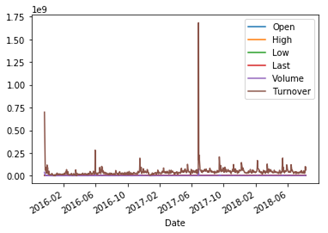The `plot()`method of`pandas` returns an Axes object. A string representation of this object is printed on the console along with the `plot()` command. To suppress this information, we can add a semicolon (;) at the end of the last statement. Alternatively, we can add a `pass` statement at the bottom of the cell. Alternatively, assigning the plotting function to a variable also suppresses the output.

### Note

By default, the `plot()` command inÂ `pandas` uses the `matplotlib` library to display graphs. If you are having errors, check to ensure this library is installed and `%matplotlib inline` is called once.

### Note

You can customize the look and feel of your charts. Further information on the `plot` command in the `pandas` DataFrame is available in the `pandas`Â documentation at https://pandas.pydata.org/pandas-docs/stable/generated/pandas.DataFrame.plot.html.

Â

### Plotting a price and volume chart

When no parameters are supplied to the `plot()` command, a line graph is plotted using all columns of the target DataFrame, on the same graph. This produces a cluttered view which does not give us much information. To effectively extract insights from this data, we can plot a financial graph of a stock with daily closing price relative to its trading volume. To facilitate this, type the following command:

```In [ ]:
prices = df['Last']
volumes = df['Volume']```

The preceding command stores our data of interest into the `closing_prices` and `volumes` variables, respectively. We can peek at the top and bottom rows of the resulting `pandas` Series data type with the `head()` and `tail()` commands:

```In [ ]:
Out[ ]:
Date
2015-11-20    18.35
2015-11-23    18.61
2015-11-24    18.80
2015-11-25    19.45
2015-11-26    19.43
Name: Last, dtype: float64

In [ ]:
volumes.tail()
Out[ ]:
Date
2018-08-03    1252024.0
2018-08-06    1126371.0
2018-08-07    1785613.0
2018-08-08    4165320.0
2018-08-09    2422470.0
Name: Volume, dtype: float64```

To find out the type of a particular variable, use the `type()` command. For example,Â `type(volumes)` produces `pandas.core.series.Series`, which tells us that the `volumes` variable is actually a `pandas`Series data type object.

Observe that data is available from 2018 all the way back to 2015. We can now plot the price and volume chart:

```In [ ]:
# The top plot consisting of daily closing prices
top = plt.subplot2grid((4, 4), (0, 0), rowspan=3, colspan=4)
top.plot(prices.index, prices, label='Last')
plt.title('ABN Last Price from 2015 - 2018')
plt.legend(loc=2)

# The bottom plot consisting of daily trading volume
bottom = plt.subplot2grid((4, 4), (3,0), rowspan=1, colspan=4)
bottom.bar(volumes.index, volumes)

plt.gcf().set_size_inches(12, 8)

This produces the following graph: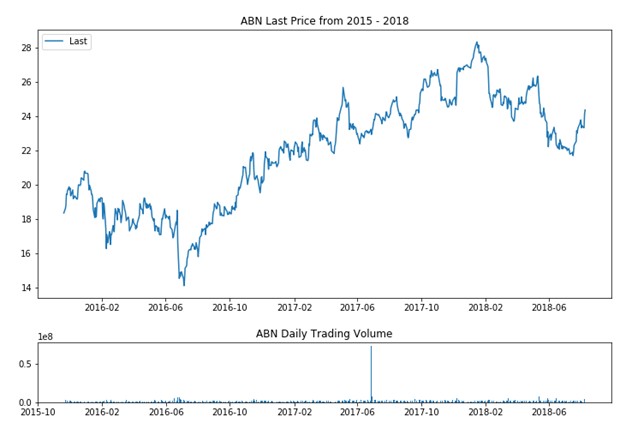On the first line, the `subplot2grid` command with the first parameter, `(4,4)`, divides the entire graph into a 4 x 4 grid. The second parameter `(0,0)` specifies that the given plot will be anchored on the top-left corner of the graph. The keyword parameter, `rowspan=3`, indicates the plot will occupy 3 of the 4 available rows on the grid, effectively as tall as 75% of the graph. The keyword parameter, `colspan=4`, indicates that the plot will occupy all 4 columns of the grid, using up all of its available width. The command returns a `matplotlib` axis object, which we will use to plot the upper portion of the graph.

On the second line, the `plot()` command renders the upper chart, with date and time values on the x axis, and prices on the y axis. In the next two lines, we specify the title of the current plot, along with a legend for the time series data placed in the upper-left corner.

Next, we perform the same actions to render the daily trading volume on the bottom chart, specifying a 1-row-by-4-column grid space anchored on the bottom-left corner of the graph.

### Note

In the `legend()` command, the `loc` keyword accepts an integer value as the location code of the legend. A value of `2` translates to an upper-left location. For a table of location codes, see the Legend documentation of `matplotlib` at https://matplotlib.org/api/legend_api.html?highlight=legend#module-matplotlib.legend.

To make our figure appear bigger, we invoke the `set_size_inches()` command to set the figure to 9 inches wide by 6 inches high, resulting in a rectangular-shaped figure. The preceding `gcf()` command simply means get current figure. Finally, the `subplots_adjust()` command with a `hspace` parameter is called to add a small amount of height between the top and bottom subplots.

### Note

The command`subplots_adjust()` tunes the subplot layout. Acceptable parameters are `left`, `right`, `bottom`, `top`, `wspace`, and `hspace`. For further information on these, see the `matplotlib` documentation at https://matplotlib.org/api/_as_gen/matplotlib.pyplot.subplots_adjust.html.

Â

### Plotting a candlestick chart

A candlestick chart is another type of popular financial chart that shows more information than just a single price. A candlestick represents a tick at each particular point of time with four important pieces of information: the open, the high, the low, and the close.

The `matplotlib.finance` module has been deprecated. Instead, we can use another package, `mpl_finance`, that consists of extracted code. To install this package, in your terminal window, type the following command:

`\$ pip install mpl-finance`

To visualize the candles more closely, we will use a subset of the ABN dataset. In the following example, we query from Quandl the daily prices for the month of July 2018 as our dataset, and plot a candlestick chart, as follows:

```In [ ]:
%matplotlib inline
import quandl
from mpl_finance import candlestick_ohlc
import matplotlib.dates as mdates
import matplotlib.pyplot as plt

quandl.ApiConfig.api_key = QUANDL_API_KEY
df_subset = quandl.get('EURONEXT/ABN',
start_date='2018-07-01',
end_date='2018-07-31')

df_subset['Date'] = df_subset.index.map(mdates.date2num)
df_ohlc = df_subset[['Date','Open', 'High', 'Low', 'Last']]

figure, ax = plt.subplots(figsize = (8,4))
formatter = mdates.DateFormatter('%Y-%m-%d')
ax.xaxis.set_major_formatter(formatter)
candlestick_ohlc(ax,
df_ohlc.values,
width=0.8,
colorup='green',
colordown='red')
plt.show()```

This produces a candlestick chart as shown in the following screenshot: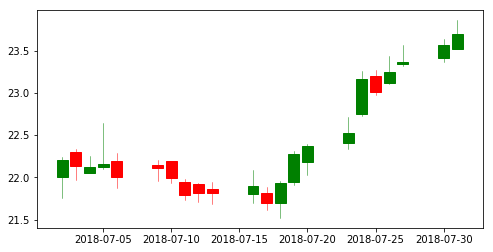### Note

You can specify the `start_date` and `end_date` parameters in the `quandl.get()` command to retrieve the dataset for the selected date range.

Prices retrieved from Quandl are placed in a variable named `df_dataset`. As theÂ plot function ofÂ `matplotlib`Â requires its own formatting, the `mdates.date2num` command converts the index values containing the date and time, and places them in a new column named `Date`.

The candlestick's date, open, high, low, and close data columns are explicitly extracted as a DataFrame in the `df_ohlc` variable. `plt.subplots()` creates a plot figure with 8 inches wide and 4 inches high. Labels along the x axis are formatted into a human-readable format.

Our data is now ready for plotting in as a candlestick chart by calling the `candlestick_ohlc()` command, with a candlestick width of 0.8 (or 80% of a full day's width). Up ticks whose close price is higher than the open price are represented in green, while down ticks, whose close price are lower than the open price, are represented in red. Finally, we add the `plt.show()` command to display the candlestick chart.

## Performing financial analytics on time series data

In this section, we will visualize some statistical properties of time series data used in financial analytics.

### Plotting returns

One of the classic measures of security performance is its returns over a prior period. A simple method for calculating returns in `pandas` is `pct_change`, where the percentage change from the previous row is computed for every row in the DataFrame.

In the following example, we use ABN stock data to plot a simple graph of daily percentage returns:

```In [ ]:
%matplotlib inline
import quandl

quandl.ApiConfig.api_key = QUANDL_API_KEY
df = quandl.get('EURONEXT/ABN.4')
daily_changes = df.pct_change(periods=1)
daily_changes.plot();```

A line plot of daily percentage returns is shown as follows: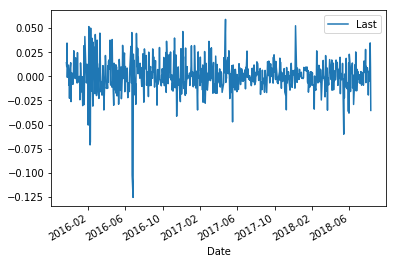In theÂ `quandl.get()`method, we postfix the ticker symbol withÂ `.4`to specify the retrieval of only the fourth column of the dataset, which contains the last prices. In the call to`pct_change`, the`period`Â argument specifies the number of periods to shift to form the percentage change, which by default is `1`.

### Note

Instead of using the postfix notation in the ticker symbol to specify the column of the dataset to download, we can pass the `column_index` parameter together with the index of the column. For example, `quandl.get('EURONEXT/ABN.4')` is the same as calling `quandl.get('EURONEXT/ABN', column_index=4)`.

### Plotting cumulative returns

To find out how our portfolio has performed, we can sum its returns over a period of time. The `cumsum` method of `pandas` returns the cumulative sum over a DataFrame.

In the following example, we plot the cumulative sum of `daily_changes` of the ABN calculated previously:

```In [ ]:
df_cumsum = daily_changes.cumsum()
df_cumsum.plot();```

This gives us the following output graph: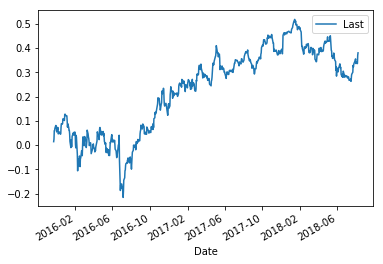### Plotting a histogram

Histograms tell us how distributed data is. In this example, we are interested in how distributed the daily returns of ABN are. We use the`hist()`method on a DataFrame with a bin size of 50:

```In [ ]:
daily_changes.hist(bins=50, figsize=(8, 4));```

The histogram output is shown as follows: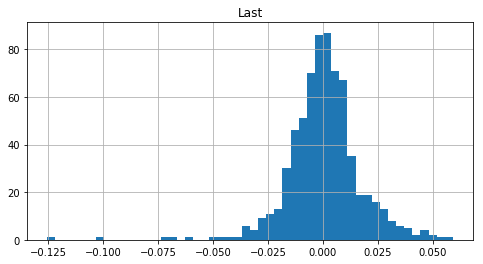When there are multiple data columns in a `pandas` DataFrame, the `hist()` method will automatically plot each histogram on its own separate plot.

We can use the `describe()` method to summarize the central tendency, dispersion, and shape of a dataset's distribution:

```In [ ]:
daily_changes.describe()
Out[ ]:
Last
count  692.000000
mean     0.000499
std      0.016701
min     -0.125527
25%     -0.007992
50%      0.000584
75%      0.008777
max      0.059123```

Â

From the histogram, the returns tend to be distributed about the mean of 0.0, or `0.000499` to be exact. Besides this miniscule skew to the right, the data appears fairly symmetrical and normally distributed. The standard deviation is `0.016701`. The percentiles tell us that 25% of the points fall below `-0.007992`, 50% below `0.000584`, and 75% below `0.008777`.

### Plotting volatility

One way of analyzing the distribution of returns is measuring its standard deviation. Standard deviation is a measure of dispersion around the mean. A high standard deviation value for past returns indicates a high historical volatility of stock price movement.

The `rolling()` method of `pandas` helps us to visualize specific time series operations over a period of time. To calculate standard deviations of the percentage change of returns in our computed ABN dataset, we use the `std()` method, which returns a DataFrame or Series object that can be used to plot a chart. The following example illustrates this:

```In [ ]:
df_filled = df.asfreq('D', method='ffill')
df_returns = df_filled.pct_change()
df_std = df_returns.rolling(window=30, min_periods=30).std()
df_std.plot();```

This gives us the following volatility plot: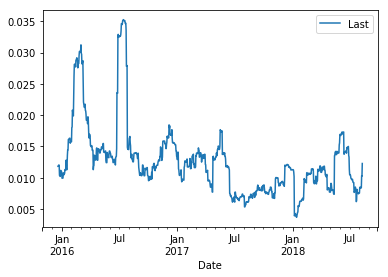Our original time series datasets exclude weekends and public holidays, which must be taken into account when using the `rolling()` method. The `df.asfreq()` command will re-index time series data on a daily frequency, creating new indexes in place of missing ones. The `method` parameter with a value of `ffill` specifies that we will propagate the last valid observation forward in place of missing values during re-indexing.

In the `rolling()` command, we specified the `window` parameter with a value of 30, which is the number of observations used for calculating the statistic. In other words, the standard deviation of each period is calculated with a sample size of 30. Since the first 30 rows do not have a sample size that is enough to calculate the standard deviation, we can exclude these rows by specifying `min_periods` as `30`.

The chosen value of 30 approximates the monthly standard deviation of returns. Note that choosing wider window periods represents less of the data being measured.

### A quantile-quantile plot

A Q-Q (quantile-quantile) plot is a probability distribution plot, where the quantiles of two distributions are plotted against each other. If the distributions are linearly related, the points in the Q-Q plot will lie along a line. Compared to histograms, Q-Q plots help us to visualize points that lie outside the line for positive and negative skews, as well as excess kurtosis.

The `probplot()` of `scipy.stats` helps us to calculate and show quantiles for a probability plot. A best-fit line for the data is also drawn. In the following example, we use the last prices of the ABN stock dataset and compute the daily percentage change for charting a Q-Q plot:

```In [ ]:
%matplotlib inline
import quandl
from scipy import stats
from scipy.stats import probplot

quandl.ApiConfig.api_key = QUANDL_API_KEY
df = quandl.get('EURONEXT/ABN.4')
daily_changes = df.pct_change(periods=1).dropna()

figure = plt.figure(figsize=(8,4))
stats.probplot(daily_changes['Last'], dist='norm', plot=ax)
plt.show();```

Â

This gives us the following Q-Q plot: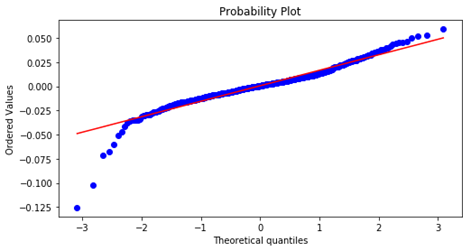When all points fall exactly along the red line, the distribution of data implies perfect correspondences to a normal distribution. Most of our data is close to being perfectly correlated between quantiles -2 and +2. Outside this range, there begin to be differences in correlation of the distribution, with more negative skews at the tails.

We pass a single Quandl code as a string object in the first parameter of the `quandl.get()` command to download a single dataset. To download multiple datasets, we can pass a list of Quandl codes.

In the following example, we are interested in the prices of three banking stocksâ€”ABN Amro, Banco Santander, and Kas Bank. The two-year prices from 2016 to 2017 are stored in the `df` variable, with only the last prices downloaded:

```In [ ]:
%matplotlib inline
import quandl

quandl.ApiConfig.api_key = QUANDL_API_KEY
df = quandl.get(['EURONEXT/ABN.4',
'EURONEXT/SANTA.4',
'EURONEXT/KA.4'],
collapse='monthly',
start_date='2016-01-01',
end_date='2017-12-31')
df.plot();```

The following plot is generated: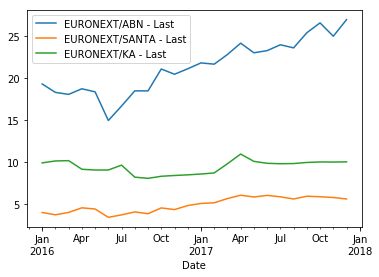### Note

By default, `quandl.get()` returns daily prices. We may also specify other types of frequency for the dataset to download. In this example, we specifiedÂ `collapse='monthly'` to download monthly prices.

### Displaying the correlation matrix

Correlation is a statistical association of how closely two variables have a linear relationship with each other. We can perform a correlation calculation on the returns of two time series datasets to give us a value between -1 and 1. A correlation value of 0 indicates that the returns of the two time series have no relation to each other. A high correlation value close to 1 indicates that the returns of the two time series data tend to move together. A low value close to -1 indicates that returns tend to move inversely in relation to each other.

In `pandas`, the `corr()` method computes the correlations between columns in its supplied DataFrame and outputs these values as a matrix. In the previous example, we have three datasets available in the DataFrame `df`. To output the correlation matrix of returns, run the following command:

```In [ ]:
df.pct_change().corr()
Out[ ]:
EURONEXT/ABN - Last ... EURONEXT/KA - Last
EURONEXT/ABN - Last               1.000000 ...           0.096238
EURONEXT/SANTA - Last             0.809824 ...           0.058095
EURONEXT/KA - Last                0.096238 ...           1.000000```

From the correlation matrix output, we can infer that the ABN Amro and Banco Santander stocks are highly correlated during the two years from 2016 to 2017 with a value of `0.809824`.

By default, the `corr()` command uses the Pearson correlation coefficient to computeÂ pairwise correlations. This is equivalent to calling `corr(method='pearson')`. Other valid values are `kendall` and `spearman` for the Kendall Tau and Spearman rank correlation coefficients, respectively.

### Plotting correlations

Visualizing correlations can also be achieved with the `rolling()` command. We will use the Last prices of ABN and SANTA on a daily basis from 2016 to 2017, from Quandl. The two datasets are downloaded to the DataFrame `df`, and its rolling correlations plotted as follows:

```In [ ]:
%matplotlib inline
import quandl

quandl.ApiConfig.api_key = QUANDL_API_KEY
df = quandl.get(['EURONEXT/ABN.4', 'EURONEXT/SANTA.4'],
start_date='2016-01-01',
end_date='2017-12-31')

df_filled = df.asfreq('D', method='ffill')
daily_changes= df_filled.pct_change()
abn_returns = daily_changes['EURONEXT/ABN - Last']
santa_returns = daily_changes['EURONEXT/SANTA - Last']
window = int(len(df_filled.index)/2)
df_corrs = abn_returns\
.rolling(window=window, min_periods=window)\
.corr(other=santa_returns)
.dropna()
df_corrs.plot(figsize=(12,8));```

The correlation plot is shown in the following screenshot: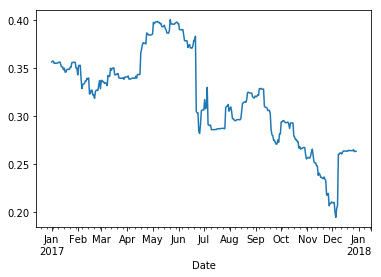The `df_filled` variable contains a DataFrame with its index re-indexed on a daily frequency basis and missing values forward-filled in preparation for the `rolling()`Â command. The DataFrame, `daily_changes`, stores the daily percentage returns, and its columns are extracted into a separate Series object as `abn_returns` and `santa_returns`, respectively. The `window` variable stores the average number of days per year in the two-year dataset. This variable is supplied into the parameters of the `rolling()` command. The parameter `window` indicates we will perform a one-year rolling correlation. The `min_periods` parameter indicates that correlation will be calculated when only the full sample size is present for calculation. In this case, there are no correlation values for the first year in the `df_corrs` dataset. Finally, the `plot()` command displays the chart of one-year rolling correlations of daily returns throughout the year of 2017.

### Simple moving averages

A common technical indicator for time series data analysis is moving averages. The `mean()` method can be used to compute the mean of values for a given window in the `rolling()` command. For example, a 5-day Simple Moving Average (SMA) is the average of prices for the last five trading days, computed daily over a time period. Similarly, we can also compute a longer term 30-day simple moving average. These two moving averages can be used together to generate crossover signals.

In the following example, we download the daily closing prices of ABN, compute the short- and long-term SMAs, and visualize them on a single plot:

```In [ ]:
%matplotlib inline
import quandl
import pandas as pd

quandl.ApiConfig.api_key = QUANDL_API_KEY
df = quandl.get('EURONEXT/ABN.4')

df_filled = df.asfreq('D', method='ffill')
df_last = df['Last']

series_short = df_last.rolling(window=5, min_periods=5).mean()
series_long = df_last.rolling(window=30, min_periods=30).mean()

df_sma = pd.DataFrame(columns=['short', 'long'])
df_sma['short'] = series_short
df_sma['long'] = series_long
df_sma.plot(figsize=(12, 8));```

Â

This produces the following plots: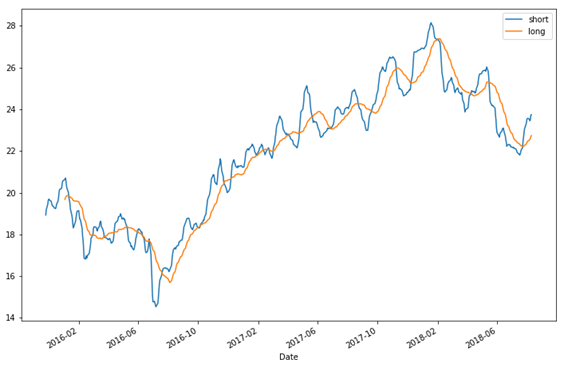We use a 5-day average for the short-term SMA and 30 days for a long-term SMA. The `min_periods` parameter is supplied to exclude the first rows that do not have sufficient sample size for computing the SMA. The `df_sma` variable is a newly-created `pandas` DataFrame for storing SMA computations. We then plot a 12-inch-by-8-inch graph. From the graph, we can see a number of points where the short-term SMA intercepts the long-term SMA. Chartists use crossovers to identify trends and generate signals. The window periods of 5 and 10 are purely suggested values; you might tweak these values to find a suitable interpretation of your own.

### Exponential moving averages

Another approach in the calculation of moving averages is the Exponential Moving Average (EMA). Recall that the simple moving average assigns equal weight to prices within a window period. However, in EMA, the most recent prices are assigned a higher weight than older prices. This weight is assigned on an exponential basis.

The `ewm()` method of the `pandas` DataFrame provides exponential weighted functions. The `span` parameter specifies the window period for the decay behavior. The same ABN dataset with EMA is plotted as follows:

```In [ ]:
%matplotlib inline
import quandl
import pandas as pd

quandl.ApiConfig.api_key = QUANDL_API_KEY
df = quandl.get('EURONEXT/ABN.4')

df_filled = df.asfreq('D', method='ffill')
df_last = df['Last']

series_short = df_last.ewm(span=5).mean()
series_long = df_last.ewm(span=30).mean()

df_sma = pd.DataFrame(columns=['short', 'long'])
df_sma['short'] = series_short
df_sma['long'] = series_long
df_sma.plot(figsize=(12, 8));```

This produces the following plot: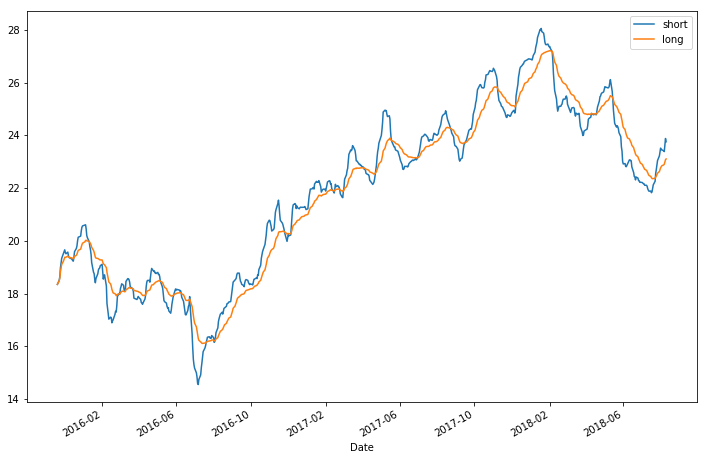The chart patterns for the SMA and EMA are largely the same. Since EMAs place a higher weighting on recent data than on older data, they are more reactive to price changes than SMAs are.

### Note

Besides varying window periods, you can experiment with combinations of SMA and EMA prices to derive more insights!

## Summary

In this chapter, we set up our working environment with Python 3.7 and used the virtual environment package to manage separate package installations. The `pip` command is a handy Python package manager that easily downloads and installs Python modules, including Jupyter, Quandl, and `pandas`. Jupyter is a browser-based interactive computational environment for executing Python code and visualizing data. With a Quandl account, we can easily obtain high-quality time series datasets. These sources of data are contributed by various data publishers. Datasets directly download into a `pandas` DataFrame object that allows us to perform financial analytics, such as plotting daily percentage returns, histograms, Q-Q plots, correlations, simple moving averages, and exponential moving averages.